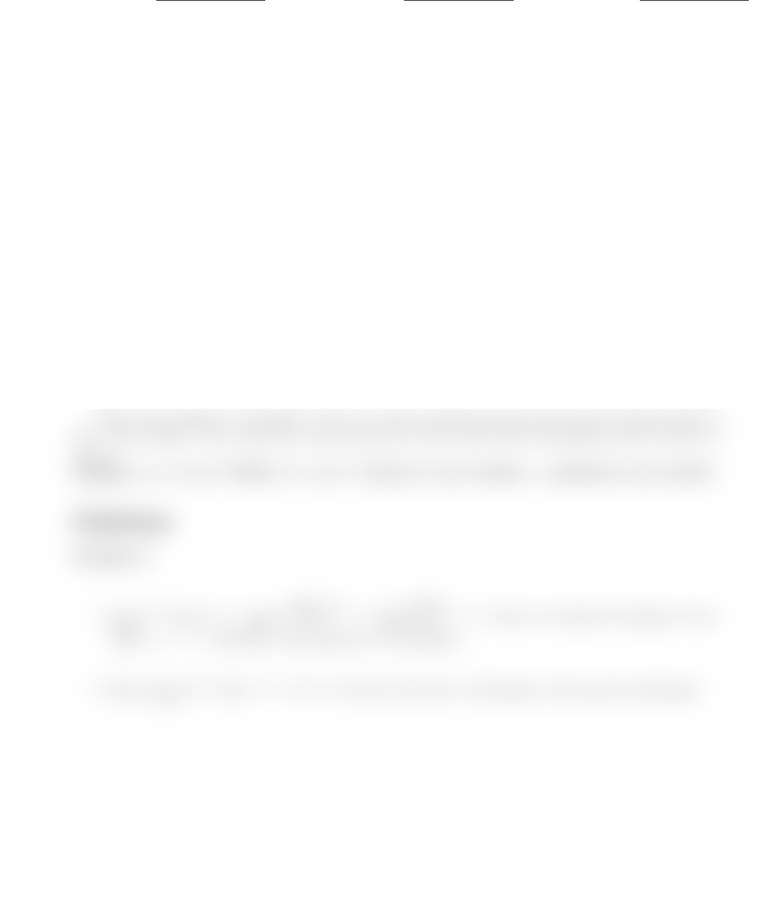Study Guides (380,000)
CA (150,000)
U of A (4,000)
MATH (300)
Quiz

# MATH 214 Quiz: Quiz 1.pdf

Department
Mathematics
Course Code
MATH214
Professor
Pierre Youssef
Study Guide
Quiz

This preview shows half of the first page. to view the full 2 pages of the document.Quiz #1.
First Name: Family Name: ID Number:
Do not speak to or communicate with other students. Calculators, textbooks, notes are not allowed.
Problem 1. (5 pt.)
1. Calculate the limit of the sequence an=en+2 sin(en). Is it convergent?
2. Is the following series convergent?
X
n=1
5en+2 sin(en)
Problem 2. (5 pt.) Is the following series convergent? If yes, ﬁnd its sum.
X
n=0
(3)n+222n
Bonus Problem. (2 pt.) I have a 2 Liters capacity empty bottle of water. The ﬁrst day, I ﬁlled
it with one liter of water. Everyday, I am allowed to add half the quantity ﬁlled the day before.
For example, the second day I would add half a liter, the third day 1/4 of a liter, etc.. I am the
only one who can add water to the bottle.
After some reﬂexion, I thought “I won’t be able to ﬁll the bottle with exactly 2 Liters before I
die”. Am I right? If not, after how many days the bottle will be full containing exactly 2 liters of
water?
Solutions
Problem 1.
1. lim
n→∞
en+2 sin(en) = lim
n→∞
e2sin(en)
en=e2lim
x0
sin(x)
x=e2, where we made the change of vari-
ables x=en. Therefore, this sequence is convergent.
2. Since lim
n→∞
5en+2 sin(en)=5e26= 0, then by the test of divergence, this series is divergent.